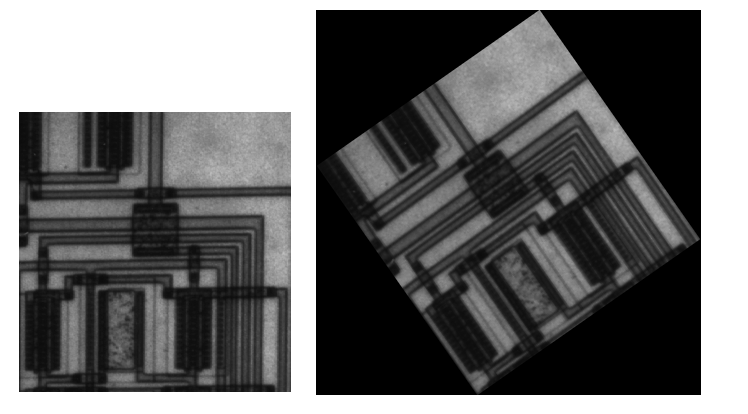Image Processing Toolbox User's GuideRotating an Image

To rotate an image, use the `imrotate` function. `imrotate` accepts two primary arguments:

• The image to be rotated
• The rotation angle

You specify the rotation angle in degrees. If you specify a positive value, `imrotate` rotates the image counterclockwise; if you specify a negative value, `imrotate` rotates the image clockwise. This example rotates the image `I` 35 degrees in the counterclockwise direction.

• ```J = imrotate(I,35);
```

As optional arguments to `imrotate`, you can also specify

Specifying the Size of the Output Image

By default, `imrotate` creates an output image large enough to include the entire original image. Pixels that fall outside the boundaries of the original image are set to 0 and appear as a black background in the output image. If you specify the text string ``crop'` as an argument, `imrotate` crops the output image to be the same size as the input image. (See the reference page for `imrotate` for an example of cropping.)

Specifying the Interpolation Method

By default, `imrotate` uses nearest-neighbor interpolation to determine the value of pixels in the output image, but you can specify other interpolation methods. This table lists the supported interpolation methods in order of complexity. See Interpolation for more information about these methods.

 Argument Value Interpolation Method `'nearest'` Nearest-neighbor interpolation (the default) `'bilinear'` Bilinear interpolation `'bicubic'` Bicubic interpolation

For example, these commands rotate an image 35° counterclockwise and use bilinear interpolation.

• ```I = imread('circuit.tif');
J = imrotate(I,35,'bilinear');
imshow(I)
figure, imshow(J)```Resizing an Image Cropping an Image